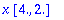Function: factrix - take out common scalar factors from a matrix or vector

Calling sequence:

factrix(M);

Parameters:

M - a matrix, vector, list or listlist, or an expression that evaluates to one of these under evalm .

Description:

If the entries of M have a scalar factor f in common, the result of this function will be f*N where f*N=M , and N has the same type as M .

In the case of an array or vector, both numerical and symbolic factors are extracted. In the case of a list or listlist, if there is no common symbolic factor a numerical factor can not be extracted (because Maple automatically distributes such factors over a list or listlist).

When some entries are fractions, everything is put over a common denominator which is extracted (except in the case noted above).

There are difficulties with factoring when floating-point coefficients are involved. This function treats any two different floats as independent (even 1.0 and 2.0).

This function is part of the Maple Advisor Database library, and must be loaded before use by the command readlib(factrix); .

Examples:

> factrix(matrix([[3/4*t^2,6*t^3],[9*q*(t+t^4),3/8*t]]));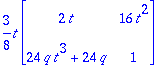> factrix(matrix([[x^2+x, x^3+x^2],
[x^3+2*x^2+x, x^3+3*x^2+3*x+1],
[w*x^2+2*w*x+w, 3*x+2+x^2]]));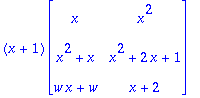> factrix(vector([15,27]));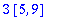> factrix(vector([1+sqrt(2),3+2*sqrt(2)]));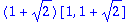> factrix([15.*x,15.*x*y]);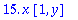> factrix([4.*x,2.*x]);